#### Thursday - February 4th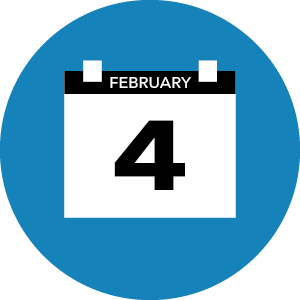### Thursday, February 4th

All times are in Central European Time (CET). Underlined authors are presenting the talks.
Click on the talk title to access the recording.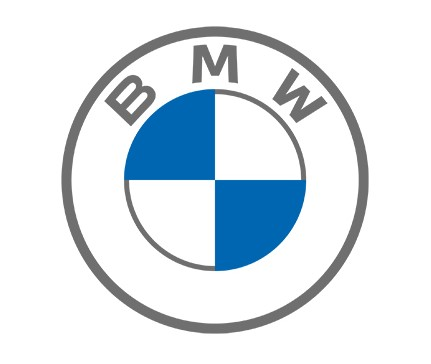Sessions on Thursday are sponsored by BMW.

### Monday | Tuesday | Wednesday | Friday

##### 10:00 | Breakfast Club - Networking

Find out who else is here and discuss via video chat in a casual atmosphere different serious (Quantum Computing: hype or hope?) and not so serious topics (What’s your favourite Bavarian dish?). Join the "Social activities" tab in Meetanyway. Topics change daily. You can join and leave tables as you wish.

### Session 1

Main stage (A)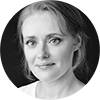##### 10:30 - 11:00 | Quantum isomorphism is equivalent to equal homomorphism counts from planar graphs

Authors: Laura Mančinska and David Roberson

Abstract

Affiliation: University of Copenhagen | Technical University of Denmark

Abstract
Over 50 years ago, Lovasz proved that two graphs are isomorphic if and only if they admit the same number of homomorphisms from any graph. Other equivalence relations on graphs, such as cospectrality or fractional isomorphism, can be characterized by equality of homomorphism counts from an appropriately chosen class of graphs. Dvorak [J. Graph Theory 2010] showed that taking this class to be the graphs of treewidth at most $k$ yields a tractable relaxation of graph isomorphism known as $k$-dimensional Weisfeiler-Leman equivalence. Together with a famous result of Cai, Furer, and Immerman [FOCS 1989], this shows that homomorphism counts from graphs of bounded treewidth do not determine a graph up to isomorphism. Dell, Grohe, and Rattan [ICALP 2018] raised the questions of whether homomorphism counts from planar graphs determine a graph up to isomorphism, and what is the complexity of the resulting relation. We answer the former in the negative by showing that the resulting relation is equivalent to the so-called quantum isomorphism [Mancinska et al, ICALP 2017]. Using this equivalence, we further resolve the latter question, showing that testing whether two graphs have the same number of homomorphisms from any planar graph is, surprisingly, an undecidable problem, and moreover is complete for the class coRE (the complement of recursively enumerable problems). Quantum isomorphism is defined in terms of a one-round, two-prover interactive proof system in which quantum provers, who are allowed to share entanglement, attempt to convince the verifier that the graphs are isomorphic. Our combinatorial proof leverages the quantum automorphism group of a graph, a notion from noncommutative mathematics.

##### 12:30 - 13:00 | Round table with Laura Mančinska

At the end of the session, you can meet with the speakers directly. Round tables take place in parallel, each speaker having their own meeting.

### Session 1A

Main stage (A)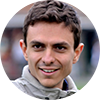11:00 - 11:30
The quantum Wasserstein distance of order 1

Authors: Giacomo De Palma, Milad Marvian, Dario Trevisan and Seth Lloyd

Abstract

Affiliations: MIT | University of New Mexico | University of Pisa | MIT

Extended abstract

Abstract
We propose a generalization of the Wasserstein distance of order 1 to the quantum states of n qudits. The proposal recovers the Hamming distance for the vectors of the canonical basis, and more generally the classical Wasserstein distance for quantum states diagonal in the canonical basis. The proposed distance is invariant with respect to permutations of the qudits and unitary operations acting on one qudit and is additive with respect to the tensor product. Our main result is a continuity bound for the von Neumann entropy with respect to the proposed distance, which significantly strengthens the best continuity bound with respect to the trace distance. We also propose a generalization of the Lipschitz constant to quantum observables. The notion of quantum Lipschitz constant allows us to compute the proposed distance with a semidefinite program. We prove a quantum version of Marton's transportation inequality and a quantum Gaussian concentration inequality for the spectrum of quantum Lipschitz observables. Moreover, we derive bounds on the contraction coefficients of shallow quantum circuits and of the tensor product of one-qudit quantum channels with respect to the proposed distance. We discuss other possible applications in quantum machine learning, quantum Shannon theory, and quantum many-body systems.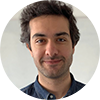Authors: Simon Becker, Nilanjana Datta, Ludovico Lami and Cambyse Rouzé

Abstract

Affiliations: University of Cambridge | University of Cambridge | Ulm University | Technische Universität München

Extended abstract

Abstract
We investigate the energy-constrained (EC) diamond norm distance between unitary channels acting on possibly infinite-dimensional quantum systems, and establish a number of results. Firstly, we prove that optimal EC discrimination between two unitary channels does not require the use of any entanglement. Extending a result by Acin, we also show that a finite number of parallel queries suffices to achieve zero error discrimination even in this EC setting. Secondly, we employ EC diamond norms to study a novel type of quantum speed limits, which apply to pairs of quantum dynamical semigroups. We expect these results to be relevant for benchmarking internal dynamics of quantum devices. Thirdly, we establish a version of the Solovay-Kitaev theorem that applies to the group of Gaussian unitaries over a finite number of modes, with the approximation error being measured with respect to the EC diamond norm relative to the photon number Hamiltonian.Authors: Gergely Bunth, Christopher Perry, Péter Vrana and Albert H. Werner

Abstract

Affiliations: Budapest University of Technology and Economics | QMath - University of Copenhagen | Budapest University of Technology and Economics | QMath - University of Copenhagen

Abstract
We study quantum dichotomies and the resource theory of asymmetric distinguishability using a generalization of Strassen's theorem on preordered semirings. We find that an asymptotic variant of relative submajorization, defined on unnormalized dichotomies, is characterized by real-valued monotones that are multiplicative under the tensor product and additive under the direct sum. These strong constraints allow us to classify and explicitly describe all such monotones, leading to a rate formula expressed as an optimization involving sandwiched Renyi divergences. As an application we give a new derivation of the strong converse error exponent in quantum hypothesis testing.

12:30 - 13:00
Round tables with Giacomo De Palma, Cambyse Rouzé, and Péter Vrana
At the end of the session, you can meet with the speakers directly. Round tables take place in parallel, each speaker having their own meeting.

### Session 1B

Stage BAuthors: Alexander Sherstov, Andrey Storozhenko and Pei Wu

Abstract

Affiliations: University of California, Los Angeles | University of California, Los Angeles | University of California, Los Angeles

Extended abstract

Abstract
We prove that for every decision tree, the absolute values of the Fourier coefficients of given order $\ell\geq1$ sum to at most $c^{\ell}\sqrt{\binom{d}{\ell}(1+\log n)^{\ell-1}},$ where $n$ is the number of variables, $d$ is the tree depth, and $c>0$ is an absolute constant. This bound is essentially tight and settles a conjecture due to Tal (arxiv 2019; FOCS 2020). The bounds prior to our work degraded rapidly with $\ell,$ becoming trivial already at $\ell=\sqrt{d}.$ As an application, we obtain, for every integer $k\geq1,$ a partial Boolean function on $n$ bits that has bounded-error quantum query complexity at most $\lceil k/2 ceil$ and randomized query complexity $\tilde{\Omega}(n^{1-1/k}).$ This separation of bounded-error quantum versus randomized query complexity is best possible, by the results of Aaronson and Ambainis (STOC 2015). Prior to our work, the best known separation was polynomially weaker: $O(1)$ versus $\Omega(n^{2/3-\epsilon})$ for any $\epsilon>0$ (Tal, FOCS 2020). As another application, we obtain an essentially optimal separation of $O(\log n)$ versus $\Omega(n^{1-\epsilon})$ for bounded-error quantum versus randomized communication complexity, for any $\epsilon>0.$ The best previous separation was polynomially weaker: $O(\log n)$ versus $\Omega(n^{2/3-\epsilon})$ (implicit in Tal, FOCS 2020).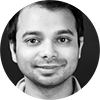Authors: Makrand Sinha and Nikhil Bansal

Abstract

Affiliations: CWI Amsterdam | CWI Amsterdam and TU Eindhoven

Extended abstract

Abstract
Aaronson and Ambainis (SICOMP 18) showed that any partial function on $N$ bits that can be computed with an advantage $\delta$ over a random guess by making $q$ quantum queries, can also be computed classically with an advantage $\delta/2$ by a randomized decision tree making ${O}_q(N^{1-\frac{1}{2q}}\delta^{-2})$ queries. Moreover, they conjectured the $k$-Forrelation problem --- a partial function that can be computed with $q = \lceil k/2 ceil$ quantum queries --- to be a suitable candidate for exhibiting such an extremal separation. We prove their conjecture by showing a tight lower bound of $\widetilde{\Omega}(N^{1-1/k})$ for the randomized query complexity of $k$-Forrelation, where the advantage $\delta = 2^{-O(k)}$. By standard amplification arguments, this gives an explicit partial function that exhibits an $O_\epsilon(1)$ vs $\Omega(N^{1-\epsilon})$ separation between bounded-error quantum and randomized query complexities, where $\epsilon>0$ can be made arbitrarily small. Our proof also gives the same bound for the closely related but non-explicit $k$-Rorrelation function introduced by Tal (FOCS 20). Our techniques rely on classical Gaussian tools, in particular, Gaussian interpolation and Gaussian integration by parts, and in fact, give a more general statement. We show that to prove lower bounds for $k$-Forrelation against a family of functions, it suffices to bound the $\ell_1$-weight of the Fourier coefficients between levels $k$ and $(k-1)k$. We also prove new interpolation and integration by parts identities that might be of independent interest in the context of rounding high-dimensional Gaussian vectors.Authors: Anurag Anshu, Shalev Ben-David and Srijita Kundu

Abstract

Affiliations: University of California, Berkeley | University of Waterloo | National University of Singapore

Extended abstract

Abstract
We investigate query-to-communication lifting theorems for models related to the quantum adversary bounds. Our results are as follows: 1. We show that the classical adversary bound lifts to a lower bound on randomized communication complexity with a constant-sized gadget. We also show that the classical adversary bound is a strictly stronger lower bound technique than the previously-lifted measure known as critical block sensitivity, making our lifting theorem one of the strongest lifting theorems for randomized communication complexity using a constant-sized gadget. 2. Turning to quantum models, we show a connection between lifting theorems for quantum adversary bounds and secure 2-party quantum computation in a certain "honest-but-curious" model. Under the assumption that such secure 2-party computation is impossible, we show that a simplified version of the positive-weight adversary bound lifts to a quantum communication lower bound using a constant-sized gadget. We also give an unconditional lifting theorem which lower bounds bounded-round quantum communication protocols. 3. Finally, we give some new results in query complexity. We show that the classical adversary and the positive-weight quantum adversary are quadratically related. We also show that the positive-weight quantum adversary is never larger than the square of the approximate degree. Both relations hold even for partial functions.

12:30 - 13:00
Round tables with Pei Wu, Makrand Sinha, and Srijita Kundu
At the end of the session, you can meet with the speakers directly. Round tables take place in parallel, each speaker having their own meeting.

### Session 1C

Stage C11:00 - 11:30
Quantum Preparation Games

Authors: Mirjam Weilenmann, Edgar A. Aguilar and Miguel Navascués

Abstract

Affiliations: IQOQI Vienna | IQOQI Vienna | IQOQI Vienna

Extended abstract

Abstract
A preparation game is a task whereby a player sequentially sends a number of quantum states to a referee, who probes each of them and announces the measurement result. The measurement setting in each round, as well as the final score of the game, are decided by the referee based on the past history of settings and measurement outcomes. Many experimental tasks in quantum information, such as entanglement quantification or magic state detection, can be cast as preparation games. In this paper, we introduce general methods to design n-round preparation games, with tight bounds on the average game scores achievable by players subject to constraints on their preparation devices. We illustrate our results by devising new adaptive measurement protocols for entanglement detection and quantification. Surprisingly, we find that the standard procedure in entanglement detection, namely, estimating n times the average value of a given entanglement witness, is in general sub-optimal for detecting the entanglement of a specific quantum state. On the contrary, there exist n-round experimental scenarios where detecting the entanglement of a known state optimally requires adaptive measurement schemes.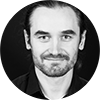Authors: Harry Buhrman, Noah Linden, Laura Mančinska, Ashley Montanaro and Maris Ozols

Abstract

Affiliations: Centrum Wiskunde & Informatica | University of Bristol | University of Copenhagen | PhaseCraft Ltd and University of Bristol | University of Amsterdam

Extended abstract

Abstract
Majority vote is a basic method for amplifying correct outcomes that is widely used in computer science and beyond. It can, for example, be used to amplify the correctness of a quantum device whose output is classical. However, when the output of a device is a quantum state, it is not apriori clear how to implement an analogous \emph{quantum} majority vote. To this end, we consider an extension of majority vote to quantum inputs and outputs: given a product state of the form $\ket{\phi_1, \phi_2, \dotsc ,\phi_n}$ where each qubit $\ket{\phi_i}$ is in one of two orthogonal states $\ket{\psi_0}$ or $\ket{\psi_1}$, output the majority state $\ket{\psi_0}$ or $\ket{\psi_1}$. We provide an optimal algorithm for this problem that achieves worst-case fidelity of $1/2 + \Theta(1/\sqrt{n})$. Under the promise that at least $2/3$ of the qubits are in the majority state, the fidelity increases to $1 - \Theta(1/n)$ and approaches one in the limit. More generally, we initiate the study of covariant and symmetric Boolean functions $f: \set{0,1^n} \to \set{0,1}$ with quantum inputs and outputs. We provide a simple linear program of size roughly $n/2$ for computing the optimal worst-case fidelity and show that a generalization of our algorithm is optimal for computing $f$. Our algorithm has complexity $O(n^4 \log n)$ where $n$ is the number of qubits.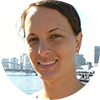Authors: Zuzana Gavorova, Matan Seidel and Yonathan Touati

Abstract

Affiliations: The Hebrew University of Jerusalem | Tel Aviv University | The Hebrew University of Jerusalem

Extended abstract

Abstract
Is a quantum algorithm capable of implementing an if-clause? Given a black-box subroutine, a d-dimensional unitary U from U(d), a quantum if-clause would correspond to applying it to an input qudit if and only if the value of a control qubit is 1. It was previously shown by Thompson et al. and Araujo et al. that implementations using single access to the oracle U are impossible. Our main result is a strong generalization of this impossibility result: we prove that there is no unitary oracle algorithm implementing control_phi(U)=|0><0|\otimes I+e^{i\phi(U)}|1><1|\otimes U, for some U-dependent phase phi(U), even if allowed any finite number of calls to U and its inverse, and even if required to only approximate the desired operator control_phi(U). Even further, there is no such postselection oracle algorithm, i.e. a unitary oracle algorithm followed by a binary success/fail measurement, that reports success and implements control_phi(U) with a nonzero probability for each U in U(d). Our proof relies on topological arguments which can be viewed as a modification of the Borsuk-Ulam theorem. Combining the topological arguments with the algorithm of Dong et al. in fact leads to an interesting dichotomy: implementing control_phi(U^m) in our model is possible if and only if the integer m is a multiple of the oracle's dimension d. Our impossibility no longer holds if the model is relaxed, either by dropping the worst-case requirement that the algorithm works for all U from U(d), or, remarkably, by allowing measurements more general than a binary postselection measurement. We observe that for both relaxations, inefficient algorithms exist, and it remains open whether efficient ones do.

12:30 - 13:00
Round tables with Mirjam Weilenmann, Maris Ozols, and Zuzana Gavorova
At the end of the session, you can meet with the speakers directly. Round tables take place in parallel, each speaker having their own meeting.

##### 13:00 - 13:15 | Digital group photo

We would like to commemorate the QIP2021 with the entire community and invite you to join us for a group photo op. This will happen in a Zoom meeting; the link will be shared in the #chit-chat_at_qip Slack channel a few minutes before the event. Join us every 5 minutes in the virtual "photo booth".

##### 14:00 on QIP TV | Lab tour "Bosonic quantum-gas microscope" with Antonio Rubio Abadal and David Wei

Join the QIP TV stage for an insight of the work done by Immanuel Bloch's group at the Max-Planck-Institute for Quantum Optics in Garching near Munich.

##### 14:30 - 15:00 | Mingling

One on one networking session - a great way to meet new people, old friends, and make valuable connections. Participants get randomly matched for one on one talks for 3 minutes. Come over in the MeetAnyway space and try it out.

### Session 2A

Main stage (A)Authors: Atul Singh Arora, Jérémie Roland and Chrysoula Vlachou

Abstract

Affiliations: Université libre de Bruxelles (ULB) and Caltech | Université libre de Bruxelles (ULB) | Université libre de Bruxelles (ULB)

Extended abstract

Abstract
Weak coin flipping (WCF) is a fundamental cryptographic primitive for two-party secure computation, where two distrustful parties need to remotely establish a shared random bit whilst having opposite preferred outcomes. It is the strongest known primitive with arbitrarily close to perfect security quantumly while classically, its security is completely compromised (unless one makes further assumptions, such as computational hardness). A WCF protocol is said to have bias \epsilon if neither party can force their preferred outcome with probability greater than 1/2 + \epsilon. Classical WCF protocols are shown to have bias 1/2, i.e., a cheating party can always force their preferred outcome. On the other hand, there exist quantum WCF protocols with arbitrarily small bias, as Mochon showed in his seminal work in 2007 [arXiv:0711.4114]. In particular, he proved the existence of a family of WCF protocols approaching bias \epsilon(k)=1/(4k + 2) for arbitrarily large k and proposed a protocol with bias 1/6. Last year, Arora, Roland and Weis presented a protocol with bias 1/10 and to go below this bias, they designed an algorithm that numerically constructs unitary matrices corresponding to WCF protocols with arbitrarily small bias [STOC'19, p.205-216]. In this work, we present new techniques which yield a fully analytical construction of WCF protocols with bias arbitrarily close to zero, thus achieving a solution that has been missing for more than a decade. Furthermore, our new techniques lead to a simplified proof of existence of WCF protocols by circumventing the non-constructive part of Mochon's proof. As an example, we illustrate the construction of a WCF protocol with bias 1/14.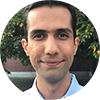Authors: Anurag Anshu, Aram Harrow and Mehdi Soleimanifar

Abstract

Affiliations: University of California, Berkeley | Massachusetts Institute of Technology | MIT

Abstract
In this work, we make a connection between two seemingly different problems. The first problem involves characterizing the properties of entanglement in the ground state of gapped local Hamiltonians, which is a central topic in quantum many-body physics. The second problem is on the quantum communication complexity of testing bipartite states with EPR assistance, a well-known question in quantum information theory. We construct a communication protocol for testing (or measuring) the ground state and use its communication complexity to reveal a new structural property for the ground state entanglement. This property, known as the entanglement spread, roughly measures the log of the ratio between the largest and the smallest Schmidt coefficients across a bipartite cut in the ground state. Our main result shows that gapped ground states possess limited entanglement spread across any cut, exhibiting an "area law" behavior. Our result applies to any interaction graph with an improved bound for the special case of lattices. This entanglement spread area law includes interaction graphs constructed in [AHL+14] that violate a generalized area law for the entanglement entropy. Our construction also provides evidence for a conjecture in physics by Li and Haldane on the entanglement spectrum of lattice Hamiltonians [LH08]. On the technical side, we use recent advances in Hamiltonian simulation algorithms along with the quantum phase estimation to give a new construction for an approximate ground space projector (AGSP) over arbitrary interaction graphs, which might be of independent interest.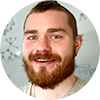Authors: Noah Shutty, Mary Wootters and Patrick Hayden

Abstract

Affiliations: Stanford University | Stanford University | Stanford University

Extended abstract

Abstract
It has long been known that the existence of certain superquantum nonlocal correlations would cause communication complexity to collapse. The absurdity of a world in which any function could be evaluated by two players with a constant amount of communication in turn provides a tantalizing way to distinguish quantum mechanics from incorrect theories of physics; the statement communication complexity is nontrivial" has even been conjectured to be a concise information-theoretic axiom for characterizing quantum mechanics. We directly address the viability of that perspective with two results. First, we exhibit a nonlocal game such that communication complexity collapses in any physical theory whose maximal winning probability exceeds the quantum value. Second, we consider the venerable CHSH game that initiated this line of inquiry. In that case, the quantum value is about 0.85 but it is known that a winning probability of approximately 0.91 would collapse communication complexity. We show that the 0.91 result is the best possible using a large class of proof strategies, suggesting that the communication complexity axiom is insufficient for characterizing CHSH correlations. Both results build on new insights about reliable classical computation. The first exploits our formalization of an equivalence between amplification and reliable computation, while the second follows from a rigorous determination of the threshold for reliable computation with formulas of noise-free XOR gates and $\epsilon$-noisy AND gates.

16:30 - 17:00
Round tables with Atul Singh Arora, Mehdi Soleimanifar, and Noah Shutty
At the end of the session, you can meet with the speakers directly. Round tables take place in parallel, each speaker having their own meeting.

### Session 2B

Stage B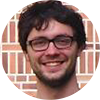Authors: Jonas Haferkamp, Felipe Montealegre-Mora, Markus Heinrich, Jens Eisert, David Gross and Ingo Roth

Abstract

Affiliations: FU Berlin | Universität Köln | Universität Köln | FU Berlin | Universität Köln | FU Berlin

Extended abstract

Abstract
Many quantum information protocols require the implementation of random unitaries. Because it takes exponential resources to produce Haar-random unitaries drawn from the full n-qubit group, one often resorts to t-designs. Unitary t-designs mimic the Haar-measure up to t-th moments. It is known that Clifford operations can implement at most 3-designs. In this work, we quantify the non-Clifford resources required to break this barrier. We find that it suffices to inject O(t^4log^2(t)log(1/e)) many non-Clifford gates into a polynomial-depth random Clifford circuit to obtain an e-approximate t-design. Strikingly, the number of non-Clifford gates required is independent of the system size -- asymptotically, the density of non-Clifford gates is allowed to tend to zero. We also derive novel bounds on the convergence time of random Clifford circuits to the t-th moment of the uniform distribution on the Clifford group. Our proofs exploit a recently developed variant of Schur-Weyl duality for the Clifford group, as well as bounds on restricted spectral gaps of averaging operators.Authors: Michal Oszmaniec, Adam Sawicki and Michal Horodecki

Abstract

Affiliations: Center for Theoretical Physics, Polish Academy of Sciences | Center for Theoretical Physics, Polish Academy of Sciences | International Centre for Theory of Quantum Technologies, University of Gdansk

Abstract
Epsilon-nets and approximate unitary t-designs are natural notions that capture properties of unitary operations relevant for numerous applications in quantum information and quantum computing. The former constitute subsets of unitary channels that are epsilon-close to any unitary channel in the diamond norm. The latter are ensembles of unitaries that (approximately) recover Haar averages of polynomials in entries of unitary channels up to order t. In this work we systematically study quantitative connections between these two notions. Specifically, we prove that, for a fixed dimension d of the Hilbert space, unitaries constituting delta-approximate t-expanders form epsilon-nets for t~(d^(5/2))/epsilon and delta~[(epsilon^(3/2))/d]^(d^2). We also show that epsilon-nets can be used to construct delta-approximate unitary t-designs for delt~epsilon*t, where the notion of approximation is based on the diamond norm. Finally, we prove that the degree of an exact unitary t-design necessary to obtain an epsilon-net must grow at least fast as 1/epsilon (for fixed dimension) and not slower than d^2 (for fixed epsilon). This shows near optimality of our result connecting t-designs and epsilon-nets. We further apply our findings in conjunction with the recent results of Varju 2013 in the context of quantum computing. First, we show that that approximate t-designs can be generated by shallow random circuits formed from a set of universal two-qudit gates in the parallel and sequential local architectures considered in Brandao-Harrow-Horodecki 2016. Importantly, our gate sets need not to be symmetric (i.e. contains gates together with their inverses) or consist of gates with algebraic entries. Second, we consider a problem of compilation of quantum gates and prove a non-constructive version of the Solovay-Kitaev theorem for general universal gate sets. Our main technical contribution is a new construction of efficient polynomial approximations to the Dirac delta in the space of quantum channels, which can be of independent interest.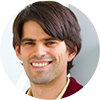16:00 - 16:30
Quantum coding with low-depth random circuits

Authors: Michael Gullans, Stefan Krastanov, David Huse, Liang Jiang and Steven Flammia

Abstract

Affiliations: Joint Center for Quantum Information and Computer Science, NIST/University of Maryland, College Park | John A. Paulson School of Engineering and Applied Sciences, Harvard University | Department of Physics, Princeton University | University of Chicago | AWS

Extended abstract

Abstract
Random quantum circuits have played a central role in establishing the computational advantages of near-term quantum computers over their conventional counterparts. Here, we use ensembles of low-depth random circuits with local connectivity in D spatial dimensions to generate quantum error-correcting codes. For random stabilizer codes and the erasure channel, we find strong evidence that a depth O(logN) random circuit is necessary and sufficient to converge (with high probability) to zero failure probability for any finite amount below the channel capacity for any D. Previous results on random circuits have only shown that O(N^1/D) depth suffices or that O(log^3 N) depth suffices for all-to-all connectivity. We then study the critical behavior of the erasure threshold in the so-called moderate deviation limit, where both the failure probability and the distance to the channel capacity converge to zero with N. We find that the requisite depth scales like O(log N) only for dimensions D=2, and that random circuits require O(N^1/2) depth for D=1. Finally, we introduce an "expurgation" algorithm that uses quantum measurements to remove logical operators that cause the code to fail by turning them into either additional stabilizers or into gauge operators in a subsystem code. With such targeted measurements, we can achieve sub-logarithmic depth in D=2 spatial dimensions below capacity without increasing the maximum weight of the check operators. We find that for any rate beneath the capacity, high-performing codes with thousands of logical qubits are achievable with depth 4-8 expurgated random circuits in D=2 dimensions. These results indicate that finite-rate quantum codes are practically relevant for near-term devices and may significantly reduce the resource requirements to achieve fault tolerance for near-term applications.

16:30 - 17:00
Round tables with Jonas Haferkamp, Michal Oszmaniec, and Michael Gullans
At the end of the session, you can meet with the speakers directly. Round tables take place in parallel, each speaker having their own meeting.

### Session 2C

Stage C15:00 - 15:30
Error mitigation with Clifford quantum-circuit data

Authors: Piotr Czarnik, Andrew Arrasmith, Patrick Coles and Lukasz Cincio

Abstract

Affiliations: Los Alamos National Laboratory | Los Alamos National Lab | Los Alamos National Laboratory | Los Alamos National Laboratory

Extended abstract

Abstract
Achieving near-term quantum advantage will require accurate estimation of quantum observables despite significant hardware noise. For this purpose, we propose a novel, scalable error-mitigation method that applies to gate-based quantum computers. The method generates training data $\{X_i^{noisy},X_i^{exact}\}$ via quantum circuits composed largely of Clifford gates, which can be efficiently simulated classically, where $X_i^{noisy}$ and $X_i^{exact}$ are noisy and noiseless observables respectively. Fitting a linear ansatz to this data then allows for the prediction of noise-free observables for arbitrary circuits. We analyze the performance of our method versus the number of qubits, circuit depth, and number of non-Clifford gates. We obtain an order-of-magnitude error reduction for a ground-state energy problem on 16 qubits in an IBMQ quantum computer and on a 64-qubit noisy simulator.15:30 - 16:00
Quantum computing enhanced computational catalysis

Authors: Guang Hao Low, Vera von Burg, Thomas Haner, Damian Steiger, Markus Reiher, Martin Roetteler and Matthias Troyer

Abstract

Affiliations: Microsoft | ETH Zurich | Microsoft | Microsoft | ETH Zurich | Microsoft | Microsoft

Abstract
The quantum computation of electronic energies can break the curse of dimensionality that plagues many-particle quantum mechanics. It is for this reason that a universal quantum computer has the potential to fundamentally change computational chemistry and materials science, areas in which strong electron correlations present severe hurdles for traditional electronic structure methods. Here, we present a state-of-the-art analysis of accurate energy measurements on a quantum computer for computational catalysis, using improved quantum algorithms with more than an order of magnitude improvement over the best previous algorithms. As a prototypical example of local catalytic chemical reactivity we consider the case of a ruthenium catalyst that can bind, activate, and transform carbon dioxide to the high-value chemical methanol. We aim at accurate resource estimates for the quantum computing steps required for assessing the electronic energy of key intermediates and transition states of its catalytic cycle. In particular, we present new quantum algorithms for double-factorized representations of the four-index integrals that can significantly reduce the computational cost over previous algorithms, and we discuss the challenges of increasing active space sizes to accurately deal with dynamical correlations. We address the requirements for future quantum hardware in order to make a universal quantum computer a successful and reliable tool for quantum computing enhanced computational materials science and chemistry, and identify open questions for further research.Authors: Isaac Kim, Eugene Tang and John Preskill

Abstract

Affiliations: The University of Sydney | California Institute of Technology | Caltech

Extended abstract

Abstract
We reconsider the black hole firewall puzzle, emphasizing that quantum error-correction, computational complexity, and pseudorandomness are crucial concepts for understanding the black hole interior. We assume that the Hawking radiation emitted by an old black hole is pseudorandom, meaning that it cannot be distinguished from a perfectly thermal state by any efficient quantum computation acting on the radiation alone. We then infer the existence of a subspace of the radiation system which we interpret as an encoding of the black hole interior. This encoded interior is entangled with the late outgoing Hawking quanta emitted by the old black hole, and is inaccessible to computationally bounded observers who are outside the black hole. Specifically, efficient operations acting on the radiation, those with quantum computational complexity polynomial in the entropy of the remaining black hole, commute with a complete set of logical operators acting on the encoded interior, up to corrections which are exponentially small in the entropy. Thus, under our pseudorandomness assumption, the black hole interior is well protected from exterior observers as long as the remaining black hole is macroscopic. On the other hand, if the radiation is not pseudorandom, an exterior observer may be able to create a firewall by applying a polynomial-time quantum computation to the radiation.

16:30 - 17:00
Round tables with Piotr Czarnik, Vera von Burg, Guang Hao Low, and Eugene Tang
At the end of the session, you can meet with the speakers directly. Round tables take place in parallel, each speaker having their own meeting.

Main stage (A)

##### 18:30 - 19:00 | Quantum Tea Time - Networking

Find out who else is here and discuss via video chat in a casual atmosphere different serious (Quantum Computing: hype or hope?) and not so serious topics (What’s your favourite Bavarian dish?). Join the "Social activities" tab in MeetAnyway. Topics change daily. You can join and leave tables as you wish.

### Industry Session

Main stage (A)##### 19:00 - 19:45 | Quantum Computer Science at Google

Speakers: Cody Jones and Ryan Babbush, Google

Abstract

Abstract
This talk will give an update regarding Google’s plans in quantum computing. We will highlight some recent results from our team and discuss some example problems where engagement with the quantum computer science community endemic to QIP will be important as the field advances. The first part of our talk will focus on quantum error-correction and the second part of our talk will focus on quantum algorithms.##### 19:45 - 20:15 | Demonstrating the capabilities of state-of-the-art quantum systems

Authors: Sarah Sheldon, IBM

Abstract

Abstract
Development of quantum hardware has accelerated in recent years and quantum systems with
tens of qubits and error rates in the 10-2 range are now reliable and accessible. The trajectory of
this development begs the question: how can we make near term systems useful without
quantum error correction? The answer is that we need new and diverse advancements in
theory, software, and hardware to allow us to approach ever harder problems. This talk will
focus in particular on using classical resources to augment the capabilities of today’s quantum
hardware, whether through classical post processing techniques or classical computations
within a quantum experiment. I will discuss recent work performed on IBM Quantum systems
to demonstrate error mitigation, benchmarking techniques for measuring progress, and
strategies for extending the range of accessible applications.

##### 20:15 - 20:45 | Round tables with Cody Jones, Ryan Babbush and Sarah Sheldon

At the end of the session, you can meet with the speakers directly. Round tables take place in parallel, each speaker having their own meeting.

##### 20:45 - 21:00 | Digital group photo

We would like to commemorate the QIP2021 with the entire community and invite you to join us for a group photo op. This will happen in a Zoom meeting; the link will be shared in the #chit-chat_at_qip Slack channel a few minutes before the event. Join us every 5 minutes in the virtual "photo booth".

##### 21:00 - 23:00 | Poster Session D

Posters in Rooms D.1 to D.4 are presenting. Visit the 3D poster rooms and use the "Video chat" button under each poster to engage with the poster representative during this time. This is linked to their poster in MeetAnyway, therefore access is possible from either platform.